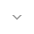belongs to Maven artifact com.android.support:support-dynamic-animation:28.0.0-alpha1

# SpringForce

``` public final class SpringForce ```
``` extends Object ``` ``` ```

 java.lang.Object ↳ android.support.animation.SpringForce

Spring Force defines the characteristics of the spring being used in the animation.

By configuring the stiffness and damping ratio, callers can create a spring with the look and feel suits their use case. Stiffness corresponds to the spring constant. The stiffer the spring is, the harder it is to stretch it, the faster it undergoes dampening.

Spring damping ratio describes how oscillations in a system decay after a disturbance. When damping ratio > 1* (i.e. over-damped), the object will quickly return to the rest position without overshooting. If damping ratio equals to 1 (i.e. critically damped), the object will return to equilibrium within the shortest amount of time. When damping ratio is less than 1 (i.e. under-damped), the mass tends to overshoot, and return, and overshoot again. Without any damping (i.e. damping ratio = 0), the mass will oscillate forever.

## Summary

### Constants

`float` `DAMPING_RATIO_HIGH_BOUNCY`

Damping ratio for a very bouncy spring.

`float` `DAMPING_RATIO_LOW_BOUNCY`

Damping ratio for a spring with low bounciness.

`float` `DAMPING_RATIO_MEDIUM_BOUNCY`

Damping ratio for a medium bouncy spring.

`float` `DAMPING_RATIO_NO_BOUNCY`

Damping ratio for a spring with no bounciness.

`float` `STIFFNESS_HIGH`

Stiffness constant for extremely stiff spring.

`float` `STIFFNESS_LOW`

Stiffness constant for a spring with low stiffness.

`float` `STIFFNESS_MEDIUM`

Stiffness constant for medium stiff spring.

`float` `STIFFNESS_VERY_LOW`

Stiffness constant for a spring with very low stiffness.

### Public constructors

``` SpringForce() ```

Creates a spring force.

``` SpringForce(float finalPosition) ```

Creates a spring with a given final rest position.

### Public methods

``` float``` ``` getDampingRatio() ```

Returns the damping ratio of the spring.

``` float``` ``` getFinalPosition() ```

Returns the rest position of the spring.

``` float``` ``` getStiffness() ```

Gets the stiffness of the spring.

``` SpringForce``` ``` setDampingRatio(float dampingRatio) ```

Spring damping ratio describes how oscillations in a system decay after a disturbance.

``` SpringForce``` ``` setFinalPosition(float finalPosition) ```

Sets the rest position of the spring.

``` SpringForce``` ``` setStiffness(float stiffness) ```

Sets the stiffness of a spring.

### Inherited methodsFrom class ``` java.lang.Object ```

## Constants

### DAMPING_RATIO_HIGH_BOUNCY

`float DAMPING_RATIO_HIGH_BOUNCY`

Damping ratio for a very bouncy spring. Note for under-damped springs (i.e. damping ratio < 1), the lower the damping ratio, the more bouncy the spring.

Constant Value: 0.2

### DAMPING_RATIO_LOW_BOUNCY

`float DAMPING_RATIO_LOW_BOUNCY`

Damping ratio for a spring with low bounciness. Note for under-damped springs (i.e. damping ratio < 1), the lower the damping ratio, the higher the bounciness.

Constant Value: 0.75

### DAMPING_RATIO_MEDIUM_BOUNCY

`float DAMPING_RATIO_MEDIUM_BOUNCY`

Damping ratio for a medium bouncy spring. This is also the default damping ratio for spring force. Note for under-damped springs (i.e. damping ratio < 1), the lower the damping ratio, the more bouncy the spring.

Constant Value: 0.5

### DAMPING_RATIO_NO_BOUNCY

`float DAMPING_RATIO_NO_BOUNCY`

Damping ratio for a spring with no bounciness. This damping ratio will create a critically damped spring that returns to equilibrium within the shortest amount of time without oscillating.

Constant Value: 1.0

### STIFFNESS_HIGH

`float STIFFNESS_HIGH`

Stiffness constant for extremely stiff spring.

Constant Value: 10000.0

### STIFFNESS_LOW

`float STIFFNESS_LOW`

Stiffness constant for a spring with low stiffness.

Constant Value: 200.0

### STIFFNESS_MEDIUM

`float STIFFNESS_MEDIUM`

Stiffness constant for medium stiff spring. This is the default stiffness for spring force.

Constant Value: 1500.0

### STIFFNESS_VERY_LOW

`float STIFFNESS_VERY_LOW`

Stiffness constant for a spring with very low stiffness.

Constant Value: 50.0

## Public constructors

### SpringForce

`SpringForce ()`

Creates a spring force. Note that final position of the spring must be set through `setFinalPosition(float)` before the spring animation starts.

### SpringForce

`SpringForce (float finalPosition)`

Creates a spring with a given final rest position.

Parameters
`finalPosition` `float`: final position of the spring when it reaches equilibrium

## Public methods

### getDampingRatio

`float getDampingRatio ()`

Returns the damping ratio of the spring.

Returns
`float` damping ratio of the spring

### getFinalPosition

`float getFinalPosition ()`

Returns the rest position of the spring.

Returns
`float` rest position of the spring

### getStiffness

`float getStiffness ()`

Gets the stiffness of the spring.

Returns
`float` the stiffness of the spring

### setDampingRatio

`SpringForce setDampingRatio (float dampingRatio)`

Spring damping ratio describes how oscillations in a system decay after a disturbance.

When damping ratio > 1 (over-damped), the object will quickly return to the rest position without overshooting. If damping ratio equals to 1 (i.e. critically damped), the object will return to equilibrium within the shortest amount of time. When damping ratio is less than 1 (i.e. under-damped), the mass tends to overshoot, and return, and overshoot again. Without any damping (i.e. damping ratio = 0), the mass will oscillate forever.

Default damping ratio is `DAMPING_RATIO_MEDIUM_BOUNCY`.

Parameters
`dampingRatio` `float`: damping ratio of the spring, it should be non-negative

Value is 0.0 or greater.

Returns
`SpringForce` the spring force that the given damping ratio is set on

Throws
`IllegalArgumentException` if the is negative.

### setFinalPosition

`SpringForce setFinalPosition (float finalPosition)`

Sets the rest position of the spring.

Parameters
`finalPosition` `float`: rest position of the spring

Returns
`SpringForce` the spring force that the given final position is set on

### setStiffness

`SpringForce setStiffness (float stiffness)`

Sets the stiffness of a spring. The more stiff a spring is, the more force it applies to the object attached when the spring is not at the final position. Default stiffness is `STIFFNESS_MEDIUM`.

Parameters
`stiffness` `float`: non-negative stiffness constant of a spring

Value is 0.0 or greater.

Returns
`SpringForce` the spring force that the given stiffness is set on

Throws
`IllegalArgumentException` if the given spring stiffness is not positive

[]
[]